# Fight Finance

#### CoursesTagsRandomAllRecentScores

This question is about the Balance of Payments. Australia's current account as a percent of nominal gross domestic product (GDP) per annum is shown in the graph below.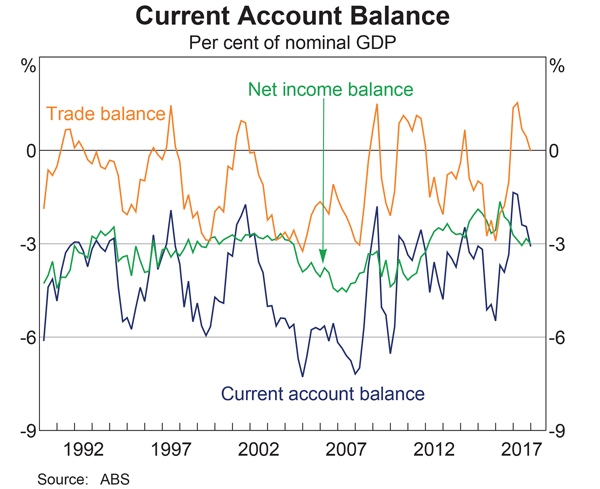Assume that all foreign and domestic assets are either debt which makes interest income or equity which makes dividend income, and vice versa for liabilities which cost interest and dividend payments, respectively.

Which of the following statements is NOT correct?

Below are four graphs of Australia's external position, published in the RBA's chart pack.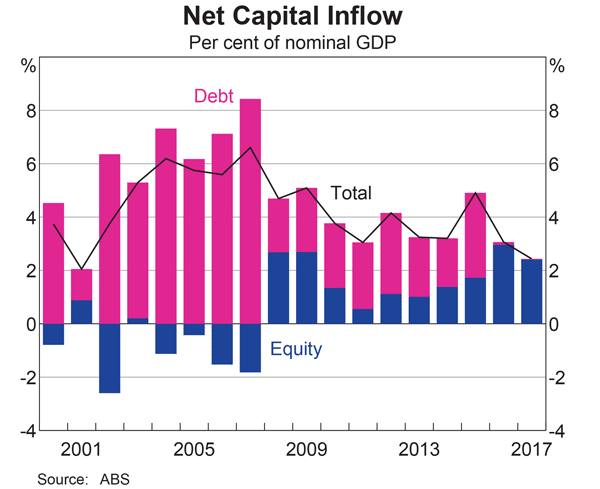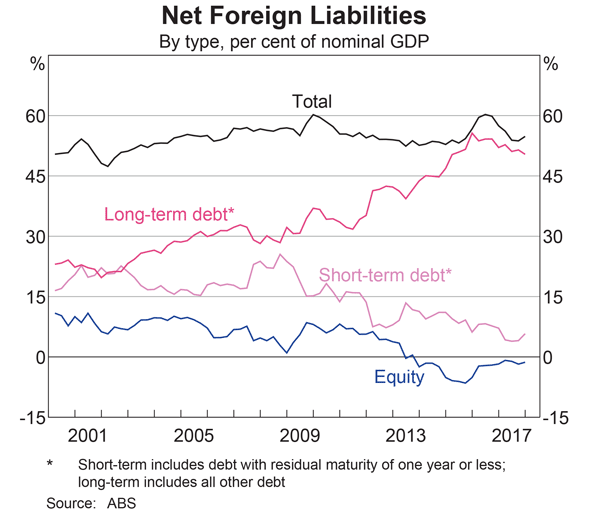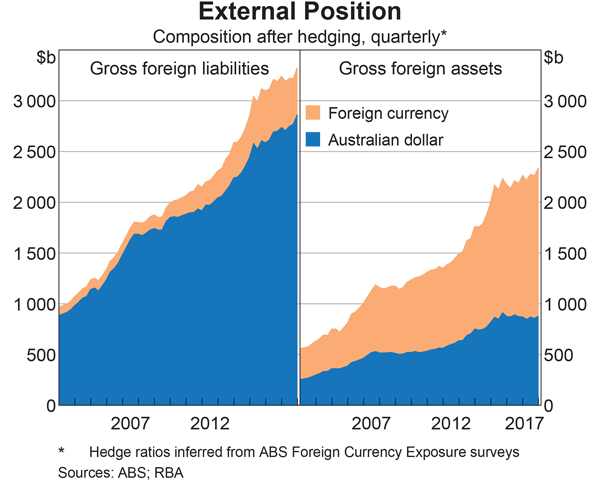Assume that all assets and liabilities are debt which makes interest income and equity which makes dividend income.

Which of the following statements is NOT correct? As at the end of 2017, Australia’s:

This question is about the Balance of Payments.

Assume that all foreign and domestic assets are either debt which makes interest income or equity which makes dividend income, and vice versa for liabilities which cost interest and dividend payments, respectively.

Which of the following statements is NOT correct?

Observe the below graph of the US current account surplus as a proportion of GDP.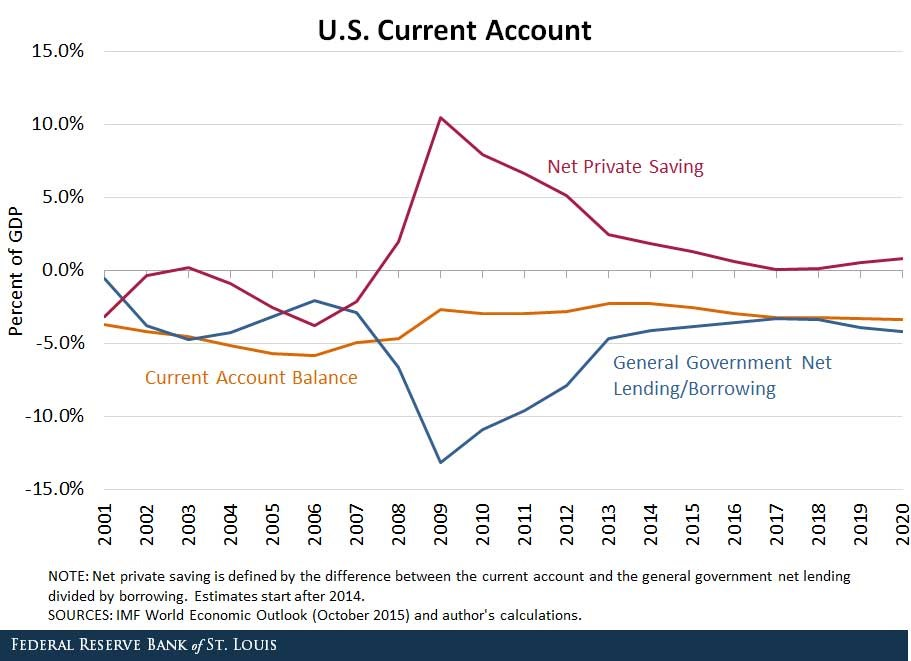Define lending as buying (or saving or investing in) debt and equity assets.

The sum of US ‘net private saving’ plus ‘net general government lending’ equals the US: